## Bamdev Mishra

### A Riemannian gossip approach to subspace learning on Grassmann manifold

#### Authors

B. Mishra, H. Kasai, P. Jawanpuria, and A. Saroop

#### Abstract

In this paper, we propose novel gossip algorithms for decentralized subspace learning problems that are modeled as finite sum problems on the Grassmann manifold. Interesting applications in this setting include low-rank matrix completion and multi-task feature learning, both of which are naturally reformulated in the considered setup. To exploit the finite sum structure, the problem is distributed among different agents and a novel cost function is proposed that is a weighted sum of the tasks handled by the agents and the communication cost among the agents. The proposed modeling approach allows local subspace learning by different agents while achieving asymptotic consensus on the global learned subspace. The resulting approach is scalable and parallelizable. Our numerical experiments show the good performance of the proposed algorithms on various benchmarks, e.g., the Netflix dataset.

• Status: Technical report, 2017. It is an extension of the earlier work [arXiv:1605.06968] to finite sum problems on the Grassmann manifold, e.g., the multitask problem. A shorter version was accepted to 9th NeurIPS workshop on optimization for machine learning (OPT2016) held at Barcelona.
• Paper: [arXiv:1705.00467]. Earlier paper [arXiv:1605.06968].
• Matlab code: Matrix completion code at [gossipMC_01May2017.zip]. Multitask code at [gossip_MTL_feat_01May2017.zip].
• Update on 01 May 2017: Uploaded the codes for matrix completion and multitask learning.
• Update on 24 Nov 2016: In the parallel code, we corrected a bug in storing the time taken by each agent for each parameter update. The bug was in using tic and toc outside a parfor loop, which added the time taken by all the agents that worked in parallel. Instead, we only needed the maximum time taken by the agents working in parallel. To accomplish this, we now use par.tic and par.toc to store the correct time taken by each agent working in parallel. This modification is based on the discussion in http://uk.mathworks.com/matlabcentral/fileexchange/27472-partictoc/content/Par.m.
• Update on 14 Aug 2016: Regularization parameter functionality added with the “options.lambda” structure.
• We provide a way to set the initial stepsize by linearizing the cost function. The stepsize is then diminished as O(1/t), where t is the iteration count.
• We provide both online and parallel implementations, and both of these implementations have both standard and preconditioned Grassmann gradient updates. The parallel implementation requires the parallel computing toolbox of Matlab.
• The performance (speed wise) of the parallel variant depends on where the algorithms are run (e.g., single machines versus multiple machines) and how well are the parallel architectures integrated with Matlab.
• All the implementations are scalable to large datasets.
• Presentation: Hiroyuki Kasai presented the work at IEICE meeting 2016, Japan.

#### Examples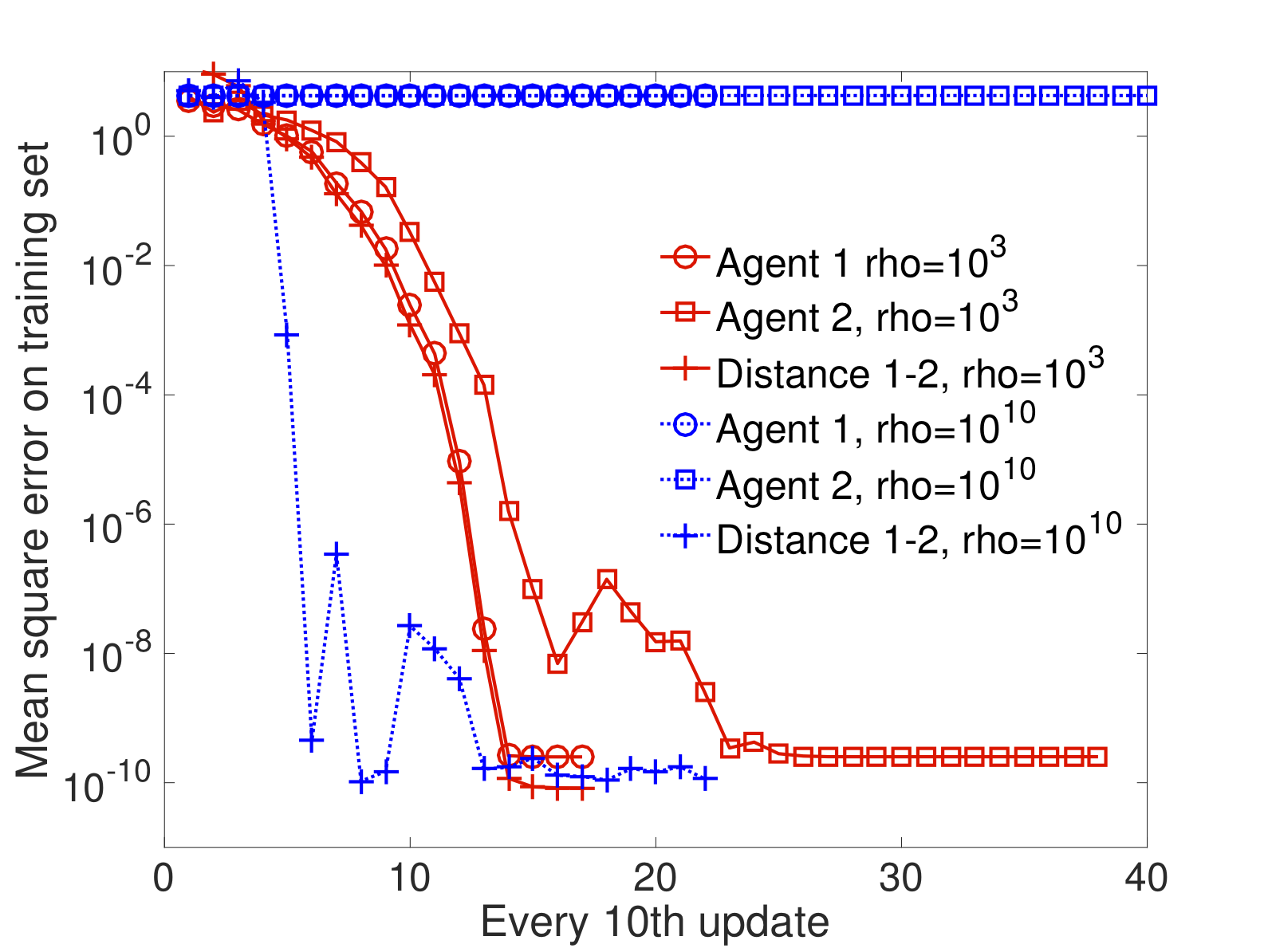Effect of ρ on arriving at consensus among six agents (only two agents are shown).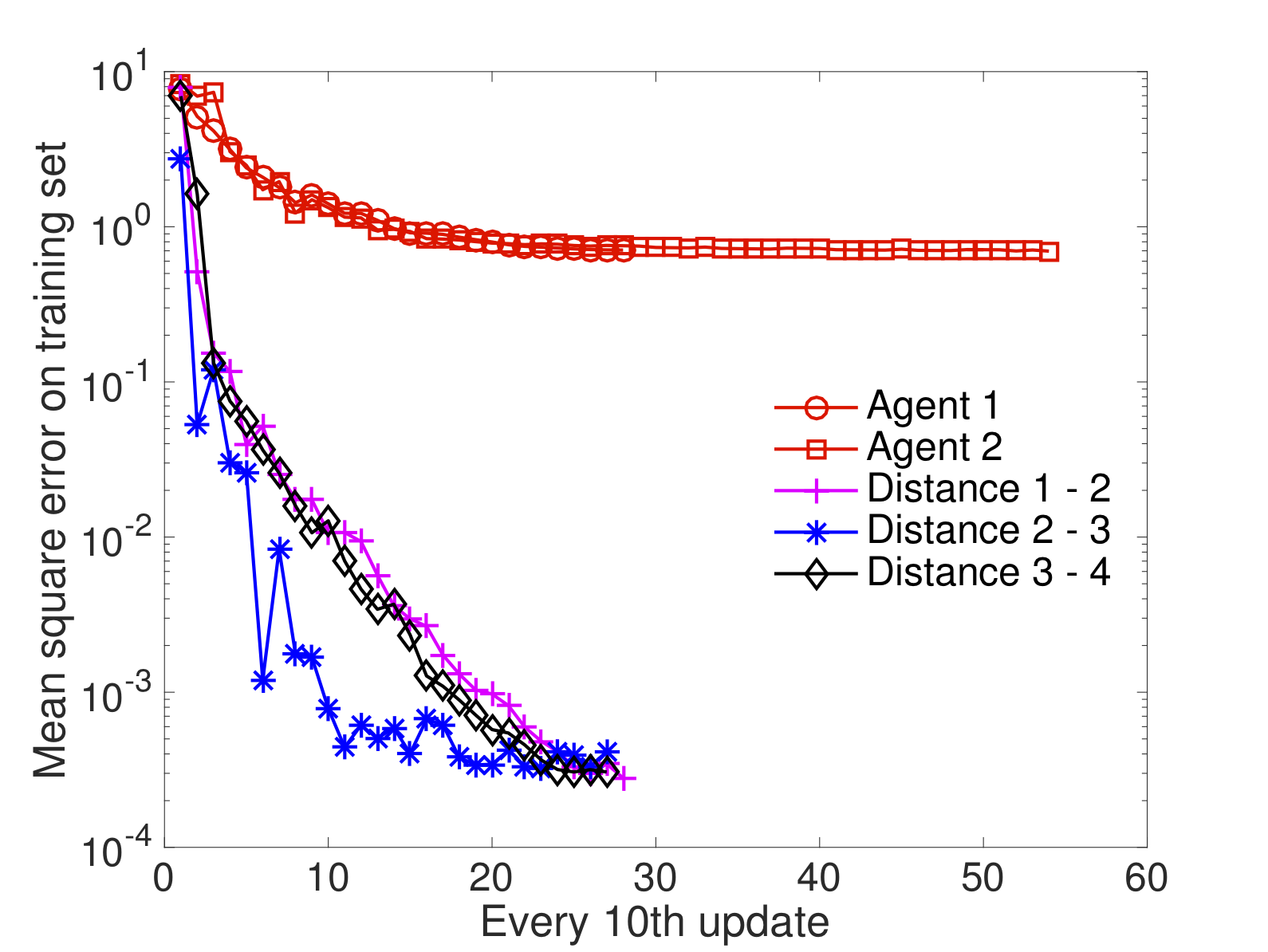MovieLens 20 M: decentralized matrix completion by four agents.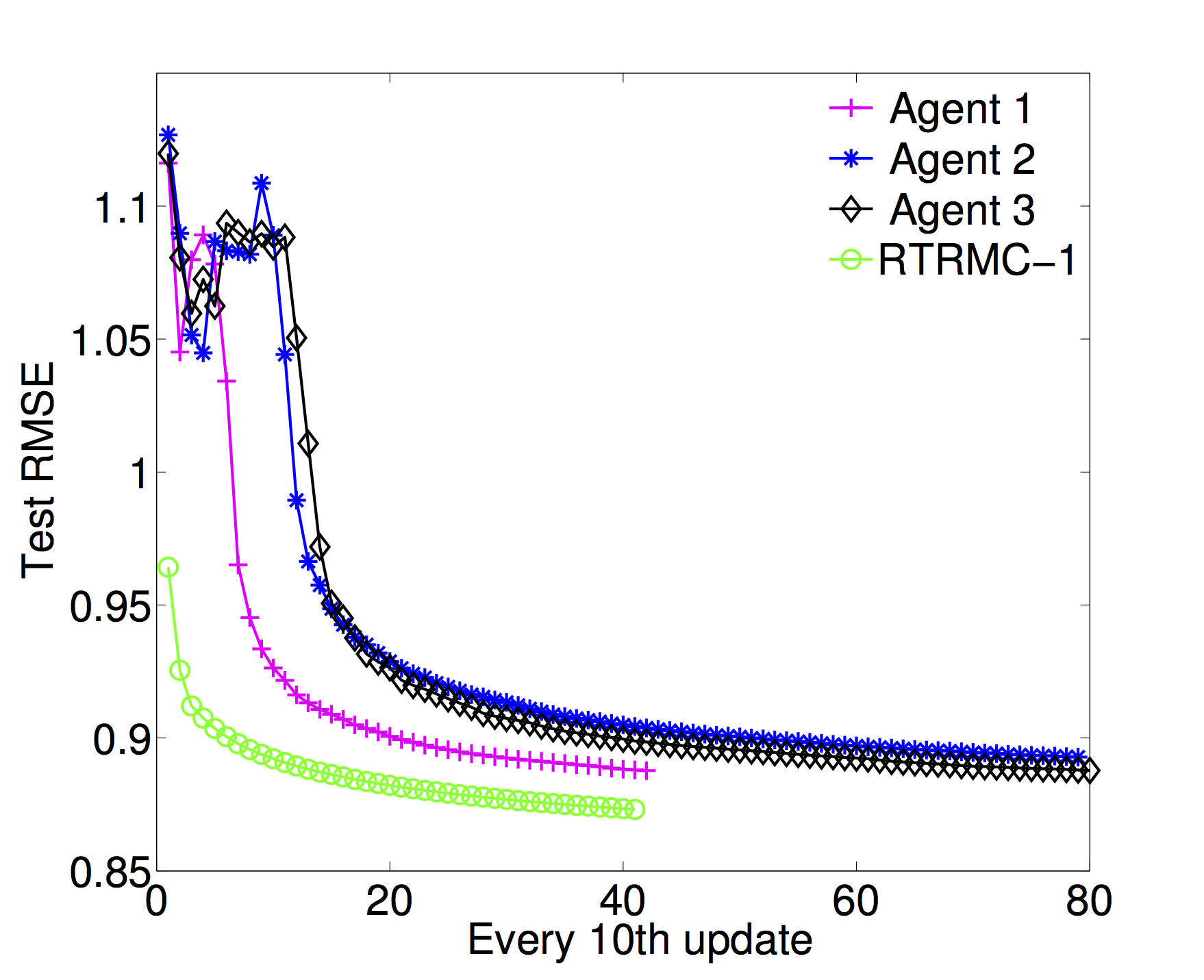Netflix: comparison of gossip with 10 agents against the batch gradient descent RTRMC.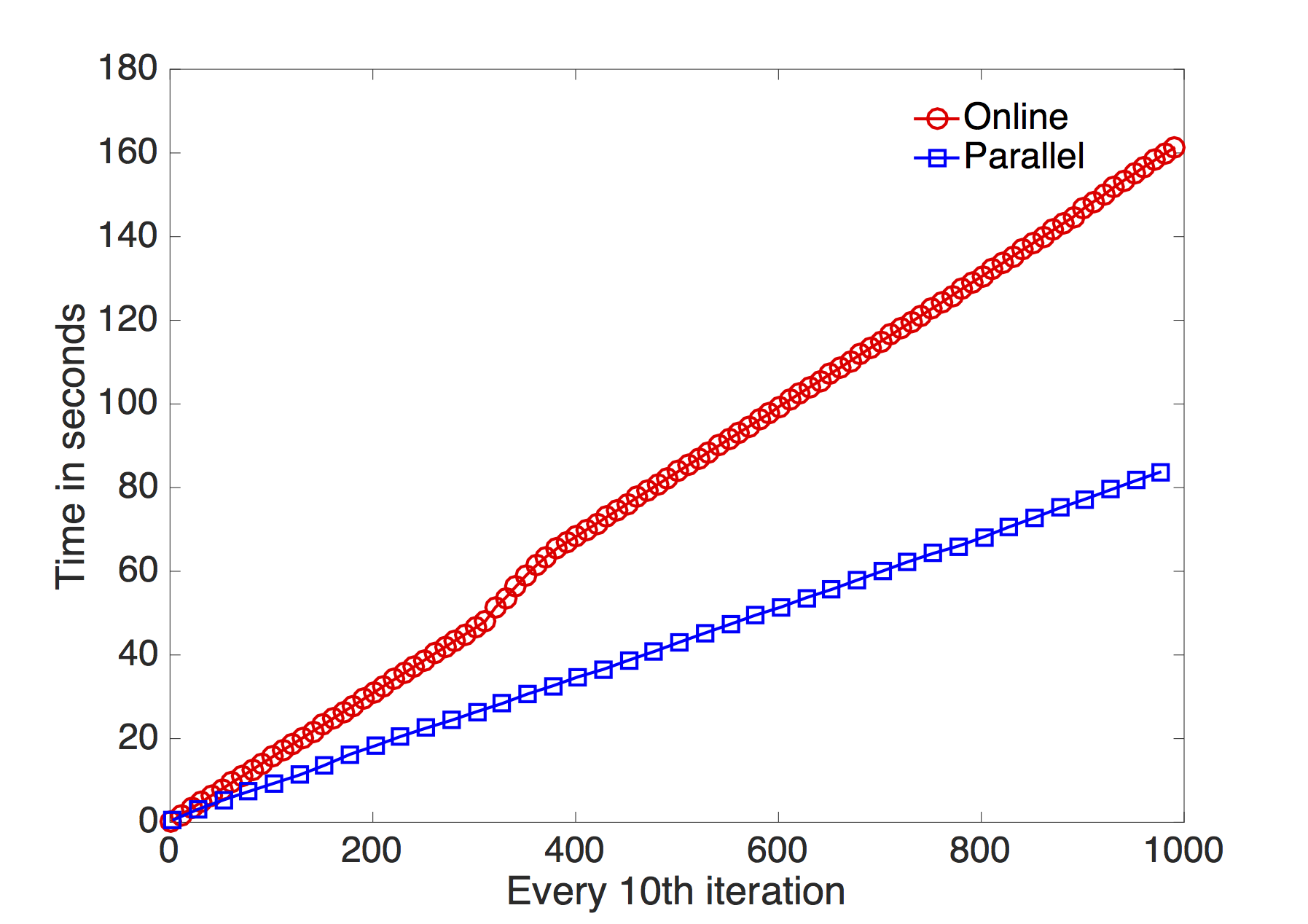Timing performance on an instance of size 10K by 100K and rank 5 with six agents. The figure shows a 2 times improvement of the parallel variant.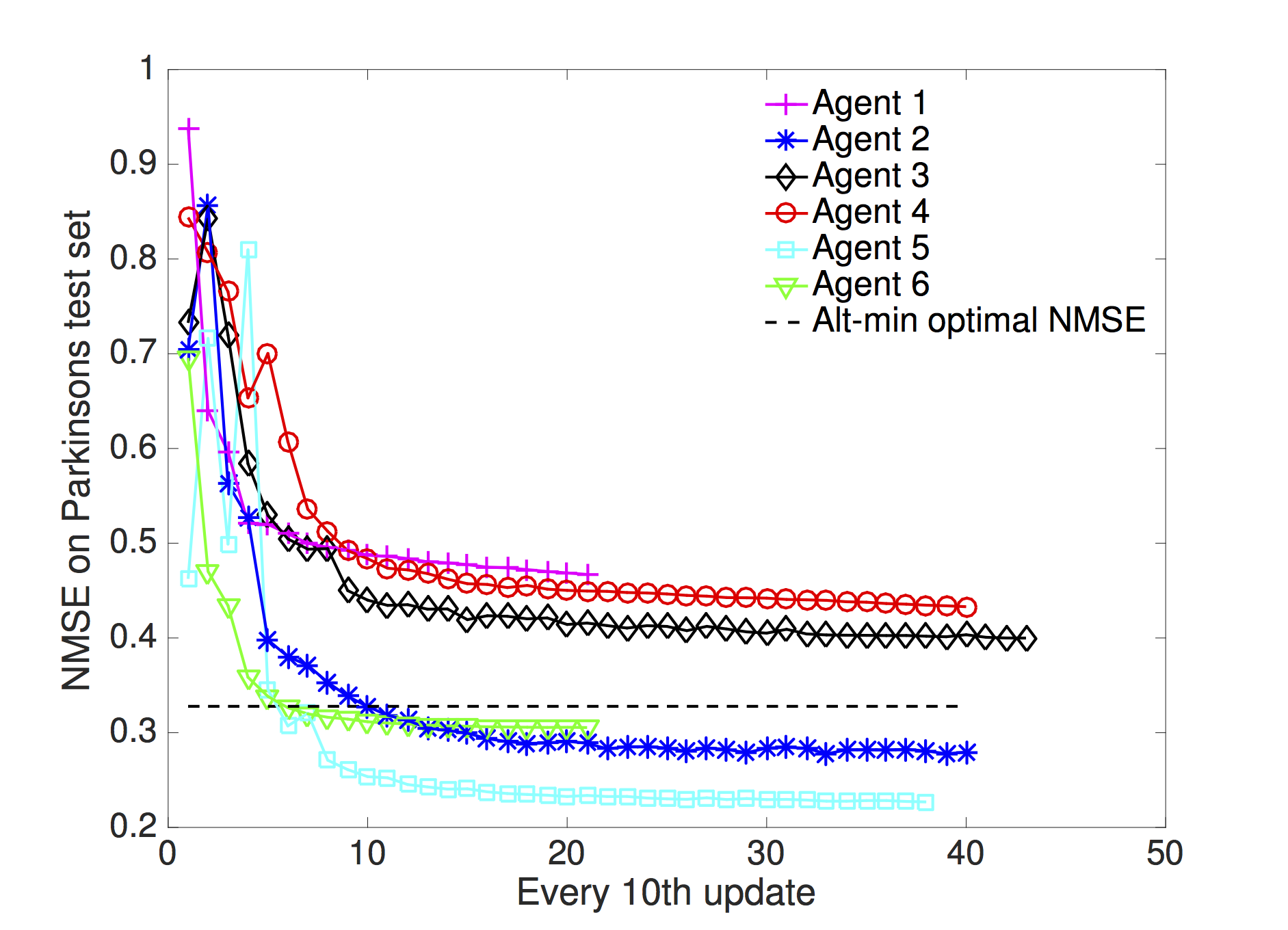Multitask learning on the Parkinsons dataset by six agents.